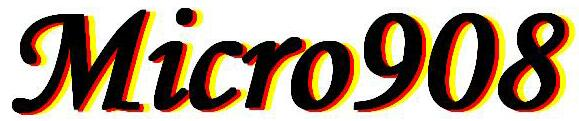COMPUTATIONS

Have you ever wondered what computations were being performed by the AA908 software inside your Micro908?  The equations used for computing SWR, Z, R, X, reactance sign, L and C, and (soon) polar notation, coax length, velocity factor, Q and more ... are all visible in the open source "calcs.asm" module, but I thought I'd describe them here in relatively plain language.

Simplified description of computations performed in the AA908 Software ...

(1) Read and correct Vf, Vr, Vz and Va
Each of the four 8-bit analog reflectometer voltages are read and "corrected" by multiplying them with the corresponding 8-bit value determined at calibration time.  This "correction" would ideally be '100' (64H), but because of channel-to-channel gain differences, it's something less than that.  This multiplication serves to correct the values (as mentioned) and also give us enough bits to work with downstream in the calculations.  Each corrected voltage is stored as a 4-byte integer number at Vf_acc, Vr_acc, Vz_acc and Va_acc (respectively).  [And remember that most computations from this point onward are done using these 4-byte accumulator representations.]

(2) Compute SWR = (Vf+Vr) / (Vf-Vr)
We actually compute SWRx100 by pre-multiplying the numerator (sum) by 100 in order to retain resolution after the divide.  If the SWR results is more than 10, we display ">10" in the display - otherwise we display x.y

(3) Compute Z = 50 (Vz/Va)
If this computation is more than 1,000, we display "Z>1K" and bypass the R and X computations.  This Z result is not displayed, but is used in the following computations for R and X.

(4) Compute Resistance R as:
R = (2500 + Z^2) * SWR / (50 * (SWR^2 + 1) )
There are lots of steps here, and each one needed to be carefully studied to ensure that there was an adequate number of bits for resolution) and well as that each did not overflow the accumulators in our custom math system.  The main change that came along in AA908 v6 software was to use double-, and in some cases triple-resolution accumulators to accommodate the large numbers coming about in these computations. In my parlance, the 4-byte accumulator described in step 1 above is single precision.  Double precision is when 8 bytes are used to represent the number, and Triple precision is when 12 bytes are used to represent the number.

(5) Compute Reactance X as:
X = SQRT ( Z_squared - R_squared )

Other operation descriptions coming soon: Reactance sign, L, C, polar notation, coax length, velocity factor and Q.

Again, look over the straightforward code in the software file "calcs.asm" for further detail on each of these steps.